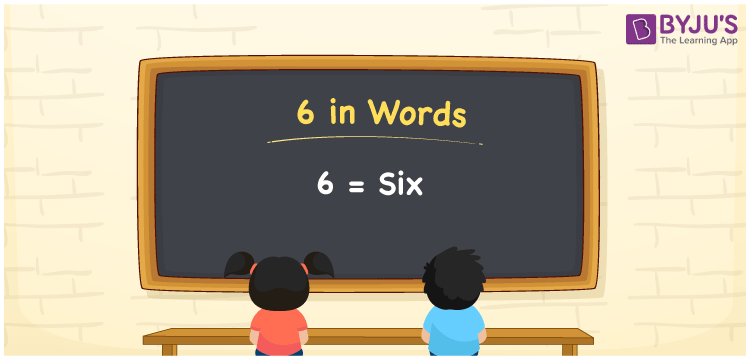# 6 in words

6 in words is written as Six. 6 represents the count or value. The article on Counting Numbers can give you an idea about count or counting. The number 6 has 1, 2, 3 and 6 as factors. It is used at multiple places and instances. For example, in case of age, we use it as “I went to London Six years back.”. Another example is, “Rohit works Six days a week”. One more example, “I have Six flowering plants”.

 6 in words Six Six in Numbers 6

## 6 in English Words## How to Write 6 in Words?

We can convert 6 to words using a place value chart. The number 6 has 1 digit. Let us check how 6 would look like in a chart that shows the place value up to 1 digit.

 Ones 6

Thus, we can write the expanded form as:

6 × One

= 6 × 1

= 6

= Six.

6 is the natural number that is succeeded by 5 and preceded by 7.

6 in words – Six.

Is 6 an odd number? – No.

Is 6 an even number? – Yes.

Is 6 a perfect square number? – No.

Is 6 a perfect cube number? – No.

Is 6 a prime number? – No

Is 6 a composite number? – Yes

## Solved Example

1. Write the number 6 in expanded form

Solution: 6 x 1

We can write 6 = 6 +0

= 6 x 1

## Frequently Asked Questions on 6 in words

Q1

### How to write 6 in words?

6 in words is written as Six.
Q2

### State if True or False. 6 is an even number and hence it is divisible by 2?

True. As 6 is an even number, it is divisible by 2. It is also divisible by 3.
Q3

### Is 6 a perfect square number?

No. 6 is not a perfect square number.